The PANEL Procedure

Two-Way Random-Effects Model

Subsections:

The specification for the two-way random-effects model is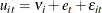As in the one-way random-effects model, the PANEL procedure provides four options for variance component estimators. Unlike the one-way random-effects model, unbalanced panels present some special concerns.

Let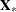and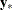be the independent and dependent variables arranged by time and by cross section within each time period. (Note that the input data set used by the PANEL procedure must be sorted by cross section and then by time within each cross section.) Let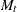be the number of cross sections observed in timeand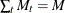. Let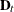be the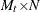matrix obtained from the
identity matrix from which rows that correspond to cross sections not observed at timehave been omitted. Consider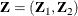where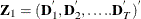and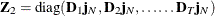.

The matrixgives the dummy variable structure for the two-way model.

For notational ease, let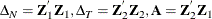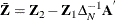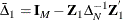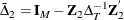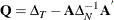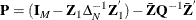Fuller and Battese’s Method

The Fuller and Battese method for estimating variance components can be obtained by setting VCOMP = FB (with the option RANTWO). FB is the default method for a RANTWO model with balanced panel. If RANTWO is requested without specifying the VCOMP= option, PROC PANEL proceeds under the Fuller and Battese method.

Following the discussion in Baltagi, Song, and Jung (2002), the Fuller and Battese method forms the estimates as follows.

The estimator of the error variance is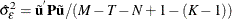where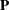is the Wansbeek and Kapteyn within estimator for unbalanced (or balanced) panel in a two-way setting.

The estimator of the error variance is the same as that in the Wansbeek and Kapteyn method.

Consider the expected values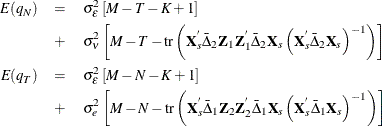Just as in the one-way case, there is always the possibility that the (estimated) variance components will be negative. In such a case, the negative components are fixed to equal zero. After substituting the group sum of the within residuals for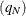, the time sums of the within residuals for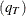, and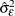, the two equations are solved for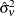and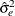.

Wansbeek and Kapteyn’s Method

The Wansbeek and Kapteyn method for estimating variance components can be obtained by setting VCOMP = WK. The following methodology, outlined in Wansbeek and Kapteyn (1989) is used to handle both balanced and unbalanced data. The Wansbeek and Kapteyn method is the default for a RANTWO model with unbalanced panel. If RANTWO is requested without specifying the VCOMP= option, PROC PANEL proceeds under the Wansbeek and Kapteyn method if the panel is unbalanced.

The estimator of the error variance iswhere the
are given by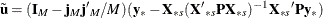if there is an intercept and by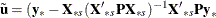if there is not.

The estimation of the variance components is performed by using a quadratic unbiased estimation (QUE) method that involves computing on quadratic forms of the residuals, equating their expected values to the realized quadratic forms, and solving for the variance components.

Let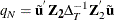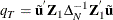The expected values are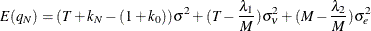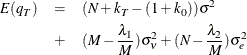where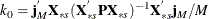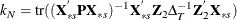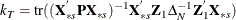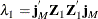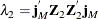The quadratic unbiased estimators for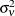and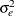are obtained by equating the expected values to the quadratic forms and solving for the two unknowns.

When the NOINT option is specified, the variance component equations change slightly. In particular, the following is true (Wansbeek and Kapteyn, 1989):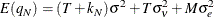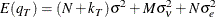Wallace and Hussain’s Method

The Wallace and Hussain method for estimating variance components can be obtained by setting VCOMP = WH. Wallace and Hussain’s method is by far the most computationally intensive. It uses the OLS residuals to estimate the variance components. In other words, the Wallace and Hussain method assumes that the following holds: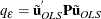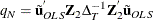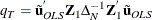Taking expectations yields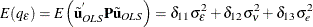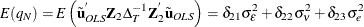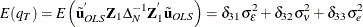where the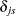constants are defined by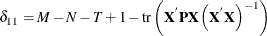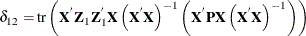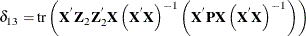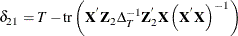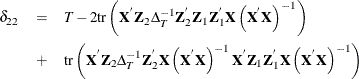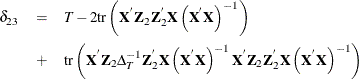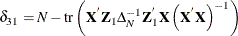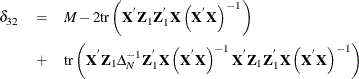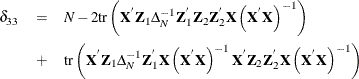The PANEL procedure solves this system for the estimates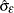,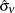, and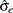. Some of the estimated variance components can be negative. Negative components are set to zero and estimation proceeds.

Nerlove’s Method

The Nerlove method for estimating variance components can be obtained with by setting VCOMP = NL.

The estimator of the error variance is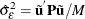The variance components for cross section and time effects are: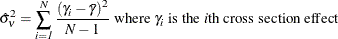and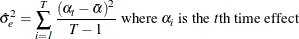Transformation and Estimation

After you calculate the estimates of the variance components, you can proceed to the final estimation. If the panel is balanced, partial mean deviations are used: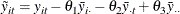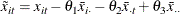Theestimates are obtained from: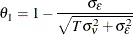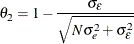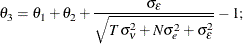With these partial deviations, PROC PANEL uses OLS on the transformed series (including an intercept if you want).

The case of an unbalanced panel is somewhat more complicated. You could naively substitute the variance components in the equation below: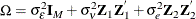After inverting the expression for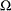, it is possible to do GLS on the data (even if the panel is unbalanced). However, the inversion ofis no small matter because the dimension is at least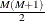.

Wansbeek and Kapteyn show that the inverse ofcan be written as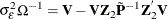with the following: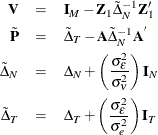Computationally, this is a much less intensive approach.

By using the inverse of the covariance matrix of the error, it becomes possible to complete GLS on the unbalanced panel.